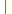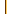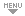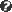# Dictionnaire Anglais-Français

French-English translation for: mathematical
ÀàÂâ...
Options | Tips | FAQ | Abbreviations

LoginSign Up
HomeAbout/ExtrasShuffleNEWVocab TrainerSubjectsUsersForumContribute!

# Translation 1 - 9 of 9FrenchEnglishADJ mathematical | more mathematical | most mathematical SYNO mathematical | numericalmath. mathématique {adj}mathematical2 Wordsmath. stat. espérance {f} mathématiquemathematical expectationmath. espérance {f}mathematical expectation [stochastic]math. logique {f} mathématiquemathematical logicmath. opération {f} mathématiquemathematical operationmath. optimisation {f} mathématiquemathematical optimisation [Br.]math. optimisation {f} mathématiquemathematical optimizationmath. optimisation {f} mathématiquemathematical programmingéduc. math. stat. études {f.pl} de mathématiquesmathematical studies» See 2 more translations for mathematical within commentsHint: Double-click next to phrase to retranslate — To translate another word just start typing!
Search time: 0.007 sec

### Contribute to the Dictionary: Add a Translation

Do you know English-French translations not listed in this dictionary? Please tell us by entering them here!
Before you submit, please have a look at the guidelines. If you can provide multiple translations, please post one by one. Make sure to provide useful source information. Important: Please also help by verifying other suggestions!

more...
English more...
Word Class more...
SubjectComment
(Source, URL)New Window